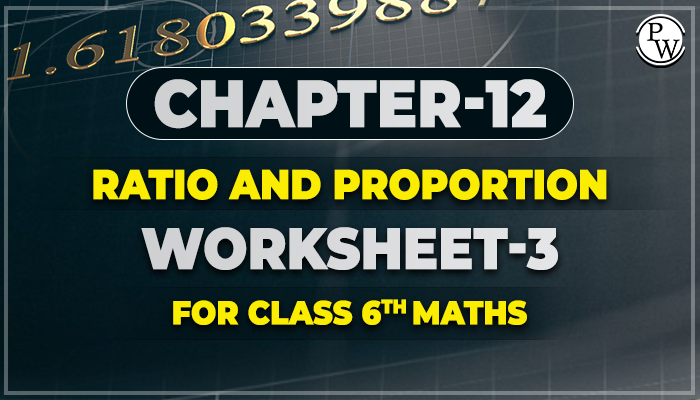# Chapter-12 Ratio and ProportionWorksheet-3 for class 6 maths

## Worksheet-3 For Class 6 Maths Chapter - Ratio and Proportion

### Ratio

It is a comparison of two quantities. The given ration p and q can be written as p : q or p/q.### How do we write ratio?

• Determine if the ratio is a fraction or a fraction to a complete.
• Count the parts once and for all if necessary.
• Connect the numbers in the scale.
• Simplify the ratio if necessary. Integer-to-integer ration are prefeered.

### Proportion

A proportion is an equality of two ratios. We write proportions to help us establish equivalent ratios and solve for unknown quantities.

### How do we use proportions?

• Write the equation using equivalent ratio.
• Connect known values ​​and use variables to represent unknown values.
• If the numerical value of one ration is a multiple of the corresponding fraction of another value, we can calculate the unknown value by multiplying another part of a given ratio by the same number.
• If the relationship between the two ratio is not clear, solve the unknown value with the varaible it represents.

### Solved Examples

Q1. In a class the number of girls in the merit list of the board examination is two times that of boys.

(a) 1 : 2

(b) 2 : 1

(c) 3 : 1

(d) 1 : 3

Ans. Correct option is (b)

The Number of girls is two times the number of boys. girls : boys = 2 : 1

Q2. The number of students passing mathematics test is 2/3 of the number that appeared.

(a) 3 : 1

(b) 1 : 2

(c) 3 : 2

(d) 2 : 3

Ans. Correct option is (d)

The number of students passing mathematics test to the number that appeared = 2 : 3

Q3. Express the ratio 186:403 in simplest form

(a) 6 : 13

(b) 5 : 8

(c) 7 : 9

(d) 13 : 6

Ans. Correct option is (a)

To express the ratio in the simplest form, divide by common number, i.e., the H.C.F. which is 31 in case of 186 and 403.

Q4. Find 8 kg to 400gm in simplest form.

(a) 1 : 50

(b) 50 : 1

(c) 20 : 1

(d) 1 : 20

Ans. Correct option is (c)

8 kg : 400gm convert kg to gm

1 kg = 1000gm

8 Kg = 8 x 1000 = 8000gm

Q5. Simplify 3 weeks to 3 days.

(a) 1 : 7

(b) 7 : 1

(c) 1 : 1

(d) 2 : 2

Ans. Correct option is (b)

Convert weeks into days, 1 week = 7 days

3 weeks = 3 x 7 = 21days

Before solving the worksheet of Physics Wallah it is highly recommend reading the theory of chapter and solve the questions given in your textbook. Do follow NCERT Solutions for class 6 Maths and NCERT Solutions for class 6 Maths Chapter Ratio and proportion prepared by Physics Wallah.

For additional information related to the subject you can check the Maths Formula section.

### Also Check

Below link consist of class 6 maths chapter-12 Ratio and Proportion download your free pdf copy from the below mentioned link of Chapter Ratio and Proportion .